# Selina Solutions Concise Maths Class 7 Chapter 21: Data Handling

Selina Solutions Concise Maths Class 7 Chapter 21 Data Handling provides students with the method of handling the given set of data. The collection of information regarding objects is called data. It is mainly of two types i.e., primary and secondary data. The data collected in its original form is called raw data. Students can access Selina Solutions Concise Maths Class 7 Chapter 21 Data Handling PDF, from the links available here.

Chapter 21 deals with tabulation of data, arithmetic or average mean, properties of mean, median, mode and bar graph. The solutions are available in both chapter wise and exercise wise PDF format, based on the needs of students..

## Selina Solutions Concise Maths Class 7 Chapter 21: Data Handling Download PDF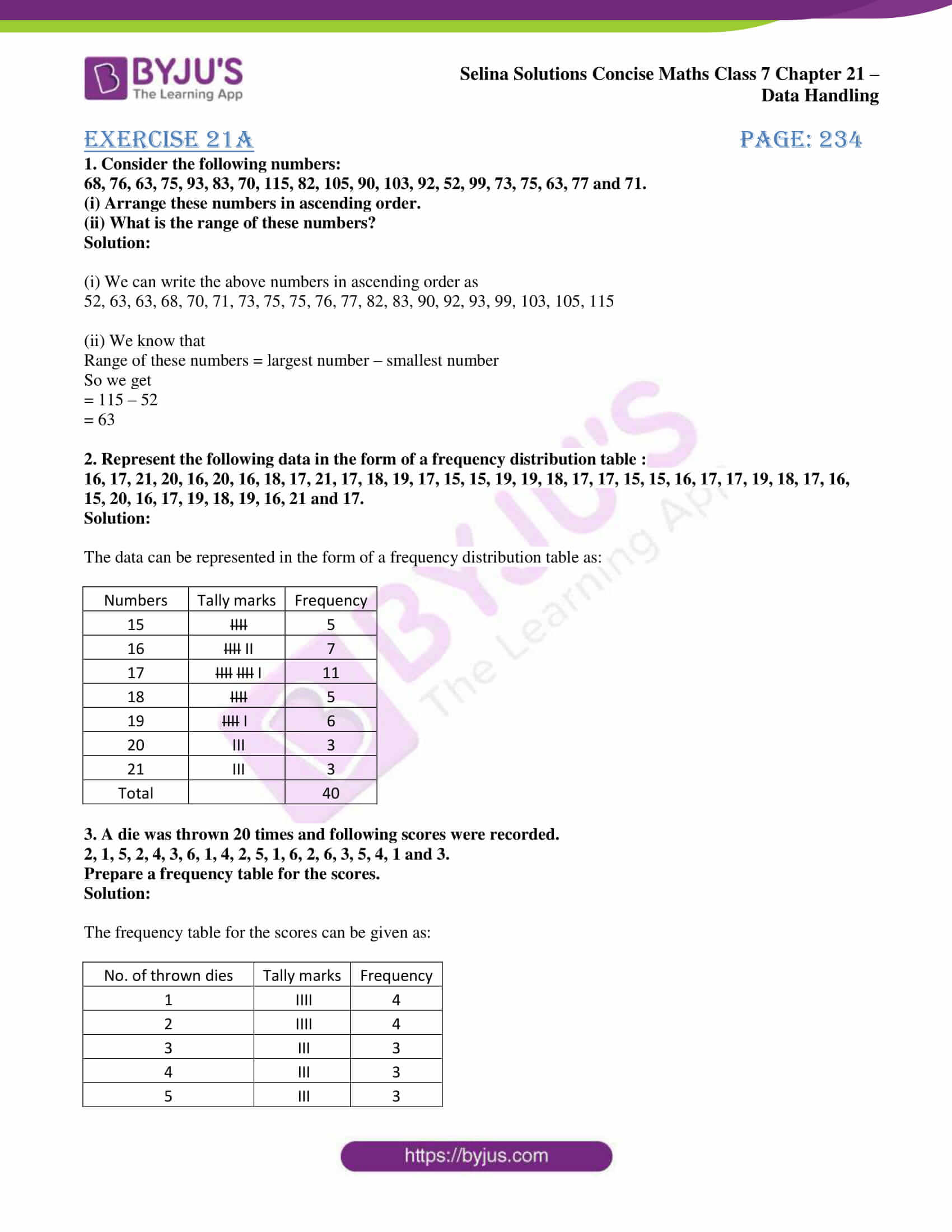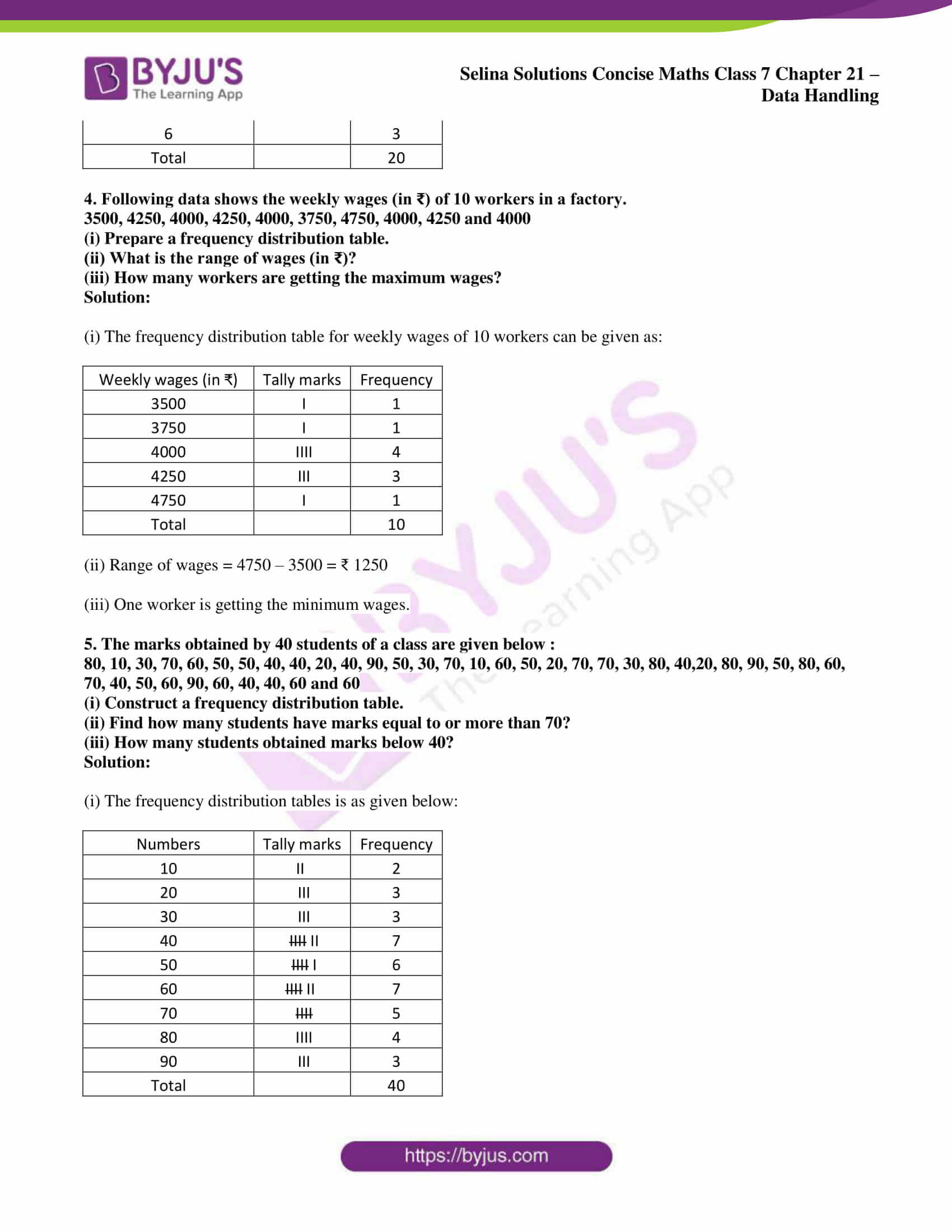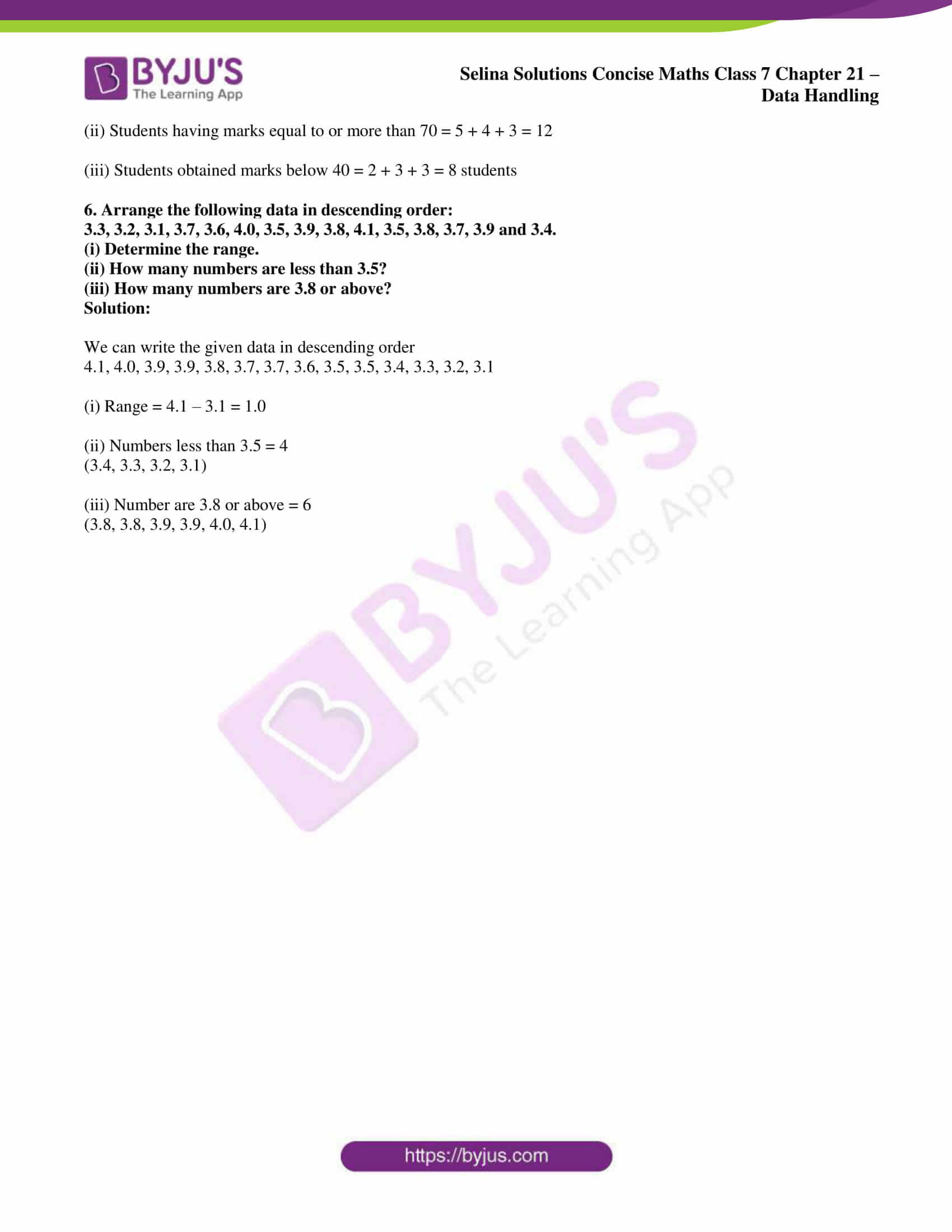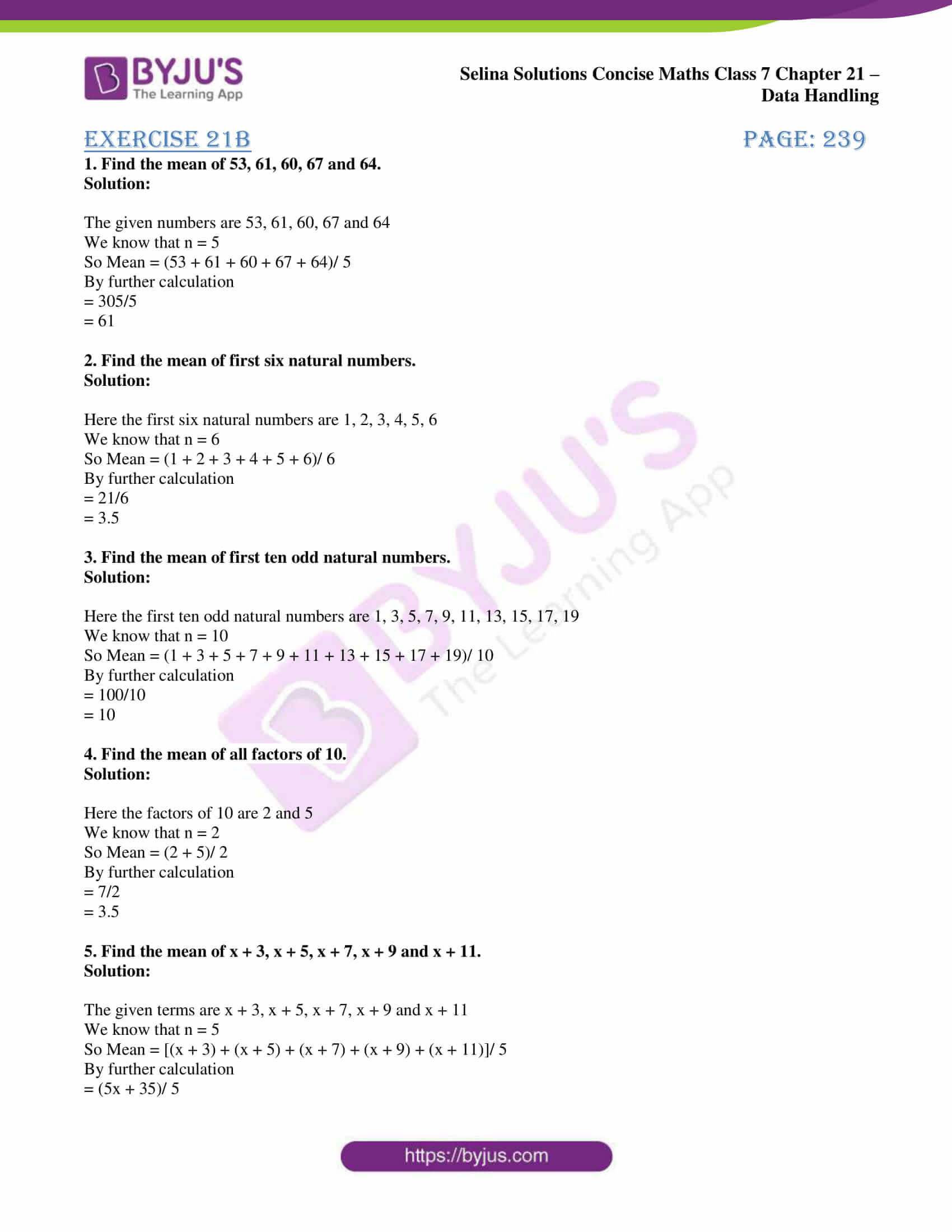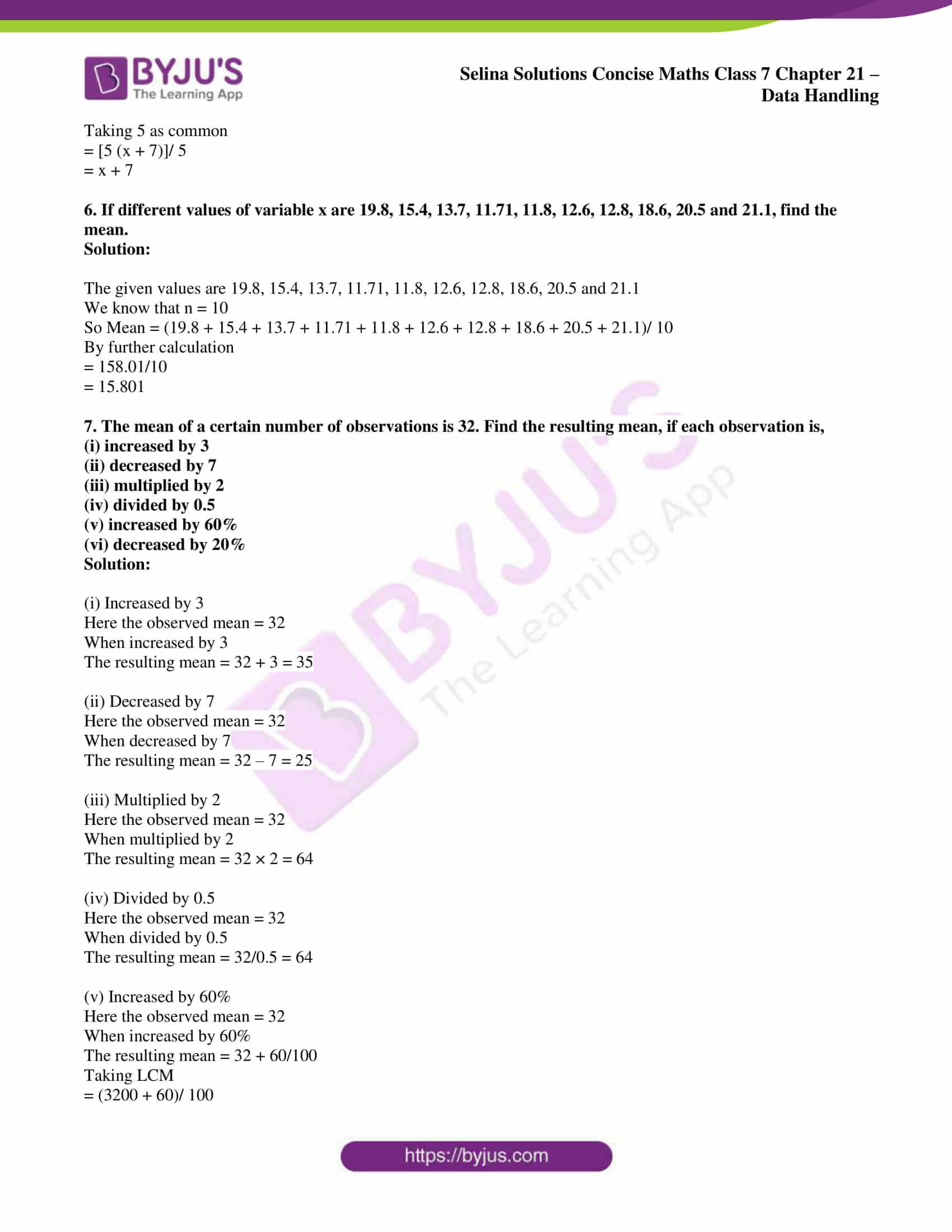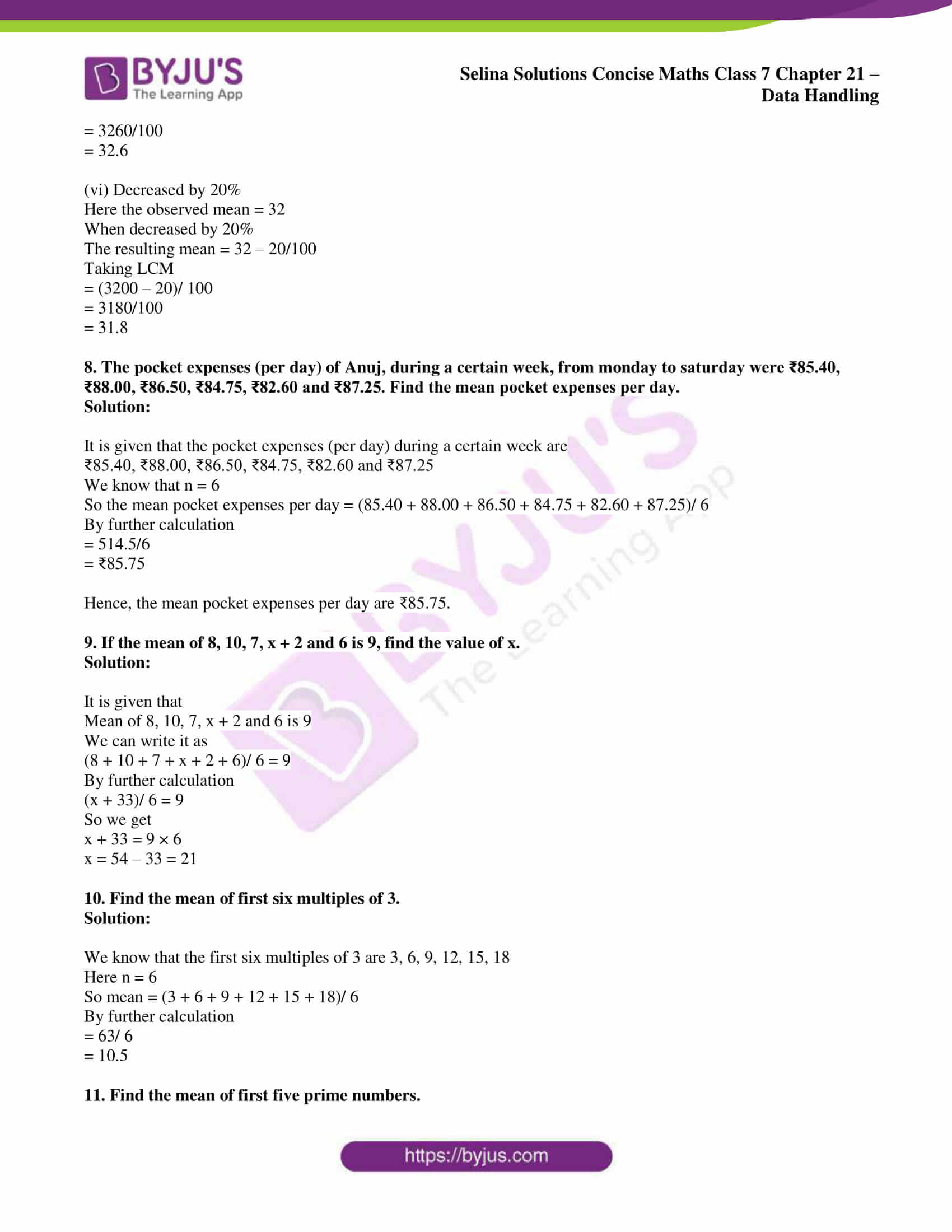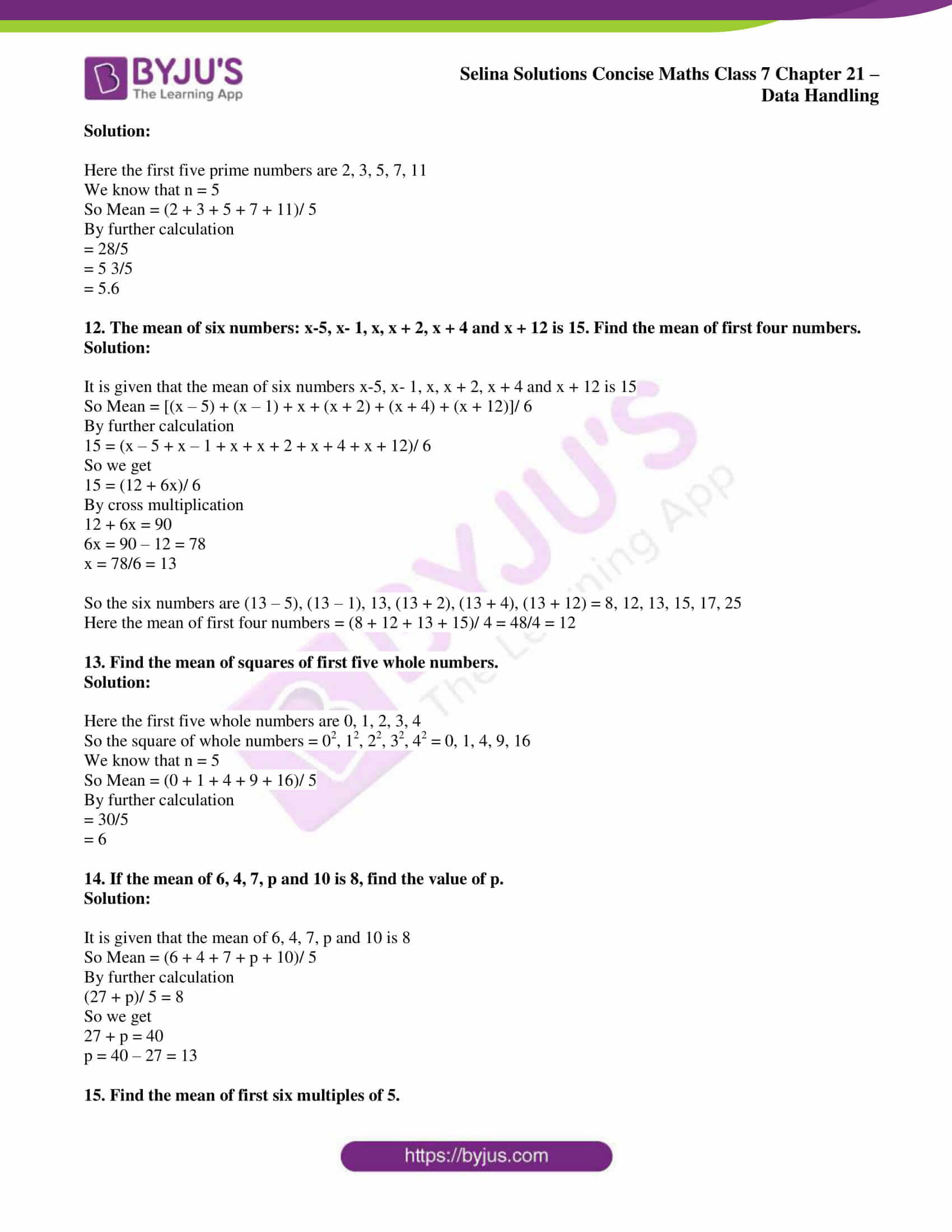## Access Selina Solutions Concise Maths Class 7 Chapter 21: Data Handling

Exercise 21A page: 234

1. Consider the following numbers:
68, 76, 63, 75, 93, 83, 70, 115, 82, 105, 90, 103, 92, 52, 99, 73, 75, 63, 77 and 71.
(i) Arrange these numbers in ascending order.
(ii) What is the range of these numbers?

Solution:

(i) We can write the above numbers in ascending order as

52, 63, 63, 68, 70, 71, 73, 75, 75, 76, 77, 82, 83, 90, 92, 93, 99, 103, 105, 115

(ii) We know that

Range of these numbers = largest number – smallest number

So we get

= 115 – 52

= 63

2. Represent the following data in the form of a frequency distribution table :

16, 17, 21, 20, 16, 20, 16, 18, 17, 21, 17, 18, 19, 17, 15, 15, 19, 19, 18, 17, 17, 15, 15, 16, 17, 17, 19, 18, 17, 16, 15, 20, 16, 17, 19, 18, 19, 16, 21 and 17.

Solution:

The data can be represented in the form of a frequency distribution table as:

 Numbers Tally marks Frequency 15 IIII 5 16 IIII II 7 17 IIII IIII I 11 18 IIII 5 19 IIII I 6 20 III 3 21 III 3 Total 40

3. A die was thrown 20 times and following scores were recorded.

2, 1, 5, 2, 4, 3, 6, 1, 4, 2, 5, 1, 6, 2, 6, 3, 5, 4, 1 and 3.

Prepare a frequency table for the scores.

Solution:

The frequency table for the scores can be given as:

 No. of thrown dies Tally marks Frequency 1 IIII 4 2 IIII 4 3 III 3 4 III 3 5 III 3 6 3 Total 20

4. Following data shows the weekly wages (in ₹) of 10 workers in a factory.

3500, 4250, 4000, 4250, 4000, 3750, 4750, 4000, 4250 and 4000

(i) Prepare a frequency distribution table.

(ii) What is the range of wages (in ₹)?

(iii) How many workers are getting the maximum wages?

Solution:

(i) The frequency distribution table for weekly wages of 10 workers can be given as:

 Weekly wages (in ₹) Tally marks Frequency 3500 I 1 3750 I 1 4000 IIII 4 4250 III 3 4750 I 1 Total 10

(ii) Range of wages = 4750 – 3500 = ₹ 1250

(iii) One worker is getting the minimum wages.

5. The marks obtained by 40 students of a class are given below :

80, 10, 30, 70, 60, 50, 50, 40, 40, 20, 40, 90, 50, 30, 70, 10, 60, 50, 20, 70, 70, 30, 80, 40,20, 80, 90, 50, 80, 60, 70, 40, 50, 60, 90, 60, 40, 40, 60 and 60

(i) Construct a frequency distribution table.

(ii) Find how many students have marks equal to or more than 70?

(iii) How many students obtained marks below 40?

Solution:

(i) The frequency distribution tables is as given below:

 Numbers Tally marks Frequency 10 II 2 20 III 3 30 III 3 40 IIII II 7 50 IIII I 6 60 IIII II 7 70 IIII 5 80 IIII 4 90 III 3 Total 40

(ii) Students having marks equal to or more than 70 = 5 + 4 + 3 = 12

(iii) Students obtained marks below 40 = 2 + 3 + 3 = 8 students

6. Arrange the following data in descending order:

3.3, 3.2, 3.1, 3.7, 3.6, 4.0, 3.5, 3.9, 3.8, 4.1, 3.5, 3.8, 3.7, 3.9 and 3.4.

(i) Determine the range.

(ii) How many numbers are less than 3.5?

(iii) How many numbers are 3.8 or above?

Solution:

We can write the given data in descending order

4.1, 4.0, 3.9, 3.9, 3.8, 3.7, 3.7, 3.6, 3.5, 3.5, 3.4, 3.3, 3.2, 3.1

(i) Range = 4.1 – 3.1 = 1.0

(ii) Numbers less than 3.5 = 4

(3.4, 3.3, 3.2, 3.1)

(iii) Number are 3.8 or above = 6

(3.8, 3.8, 3.9, 3.9, 4.0, 4.1)

Exercise 21B page: 239

1. Find the mean of 53, 61, 60, 67 and 64.

Solution:

The given numbers are 53, 61, 60, 67 and 64

We know that n = 5

So Mean = (53 + 61 + 60 + 67 + 64)/ 5

By further calculation

= 305/5

= 61

2. Find the mean of first six natural numbers.

Solution:

Here the first six natural numbers are 1, 2, 3, 4, 5, 6

We know that n = 6

So Mean = (1 + 2 + 3 + 4 + 5 + 6)/ 6

By further calculation

= 21/6

= 3.5

3. Find the mean of first ten odd natural numbers.

Solution:

Here the first ten odd natural numbers are 1, 3, 5, 7, 9, 11, 13, 15, 17, 19

We know that n = 10

So Mean = (1 + 3 + 5 + 7 + 9 + 11 + 13 + 15 + 17 + 19)/ 10

By further calculation

= 100/10

= 10

4. Find the mean of all factors of 10.

Solution:

Here the factors of 10 are 2 and 5

We know that n = 2

So Mean = (2 + 5)/ 2

By further calculation

= 7/2

= 3.5

5. Find the mean of x + 3, x + 5, x + 7, x + 9 and x + 11.

Solution:

The given terms are x + 3, x + 5, x + 7, x + 9 and x + 11

We know that n = 5

So Mean = [(x + 3) + (x + 5) + (x + 7) + (x + 9) + (x + 11)]/ 5

By further calculation

= (5x + 35)/ 5

Taking 5 as common

= [5 (x + 7)]/ 5

= x + 7

6. If different values of variable x are 19.8, 15.4, 13.7, 11.71, 11.8, 12.6, 12.8, 18.6, 20.5 and 21.1, find the mean.

Solution:

The given values are 19.8, 15.4, 13.7, 11.71, 11.8, 12.6, 12.8, 18.6, 20.5 and 21.1

We know that n = 10

So Mean = (19.8 + 15.4 + 13.7 + 11.71 + 11.8 + 12.6 + 12.8 + 18.6 + 20.5 + 21.1)/ 10

By further calculation

= 158.01/10

= 15.801

7. The mean of a certain number of observations is 32. Find the resulting mean, if each observation is,

(i) increased by 3

(ii) decreased by 7

(iii) multiplied by 2

(iv) divided by 0.5

(v) increased by 60%

(vi) decreased by 20%

Solution:

(i) Increased by 3
Here the observed mean = 32

When increased by 3

The resulting mean = 32 + 3 = 35

(ii) Decreased by 7
Here the observed mean = 32

When decreased by 7

The resulting mean = 32 – 7 = 25

(iii) Multiplied by 2
Here the observed mean = 32

When multiplied by 2

The resulting mean = 32 × 2 = 64

(iv) Divided by 0.5
Here the observed mean = 32

When divided by 0.5

The resulting mean = 32/0.5 = 64

(v) Increased by 60%
Here the observed mean = 32

When increased by 60%

The resulting mean = 32 + 60/100

Taking LCM

= (3200 + 60)/ 100

= 3260/100

= 32.6

(vi) Decreased by 20%

Here the observed mean = 32

When decreased by 20%

The resulting mean = 32 – 20/100

Taking LCM

= (3200 – 20)/ 100

= 3180/100

= 31.8

8. The pocket expenses (per day) of Anuj, during a certain week, from monday to saturday were ₹85.40, ₹88.00, ₹86.50, ₹84.75, ₹82.60 and ₹87.25. Find the mean pocket expenses per day.

Solution:

It is given that the pocket expenses (per day) during a certain week are

₹85.40, ₹88.00, ₹86.50, ₹84.75, ₹82.60 and ₹87.25

We know that n = 6

So the mean pocket expenses per day = (85.40 + 88.00 + 86.50 + 84.75 + 82.60 + 87.25)/ 6

By further calculation

= 514.5/6

= ₹85.75

Hence, the mean pocket expenses per day are ₹85.75.

9. If the mean of 8, 10, 7, x + 2 and 6 is 9, find the value of x.

Solution:

It is given that

Mean of 8, 10, 7, x + 2 and 6 is 9

We can write it as

(8 + 10 + 7 + x + 2 + 6)/ 6 = 9

By further calculation

(x + 33)/ 6 = 9

So we get

x + 33 = 9 × 6

x = 54 – 33 = 21

10. Find the mean of first six multiples of 3.

Solution:

We know that the first six multiples of 3 are 3, 6, 9, 12, 15, 18

Here n = 6

So mean = (3 + 6 + 9 + 12 + 15 + 18)/ 6

By further calculation

= 63/ 6

= 10.5

11. Find the mean of first five prime numbers.

Solution:

Here the first five prime numbers are 2, 3, 5, 7, 11

We know that n = 5

So Mean = (2 + 3 + 5 + 7 + 11)/ 5

By further calculation

= 28/5

= 5 3/5

= 5.6

12. The mean of six numbers: x-5, x- 1, x, x + 2, x + 4 and x + 12 is 15. Find the mean of first four numbers.

Solution:

It is given that the mean of six numbers x-5, x- 1, x, x + 2, x + 4 and x + 12 is 15

So Mean = [(x – 5) + (x – 1) + x + (x + 2) + (x + 4) + (x + 12)]/ 6

By further calculation

15 = (x – 5 + x – 1 + x + x + 2 + x + 4 + x + 12)/ 6

So we get

15 = (12 + 6x)/ 6

By cross multiplication

12 + 6x = 90

6x = 90 – 12 = 78

x = 78/6 = 13

So the six numbers are (13 – 5), (13 – 1), 13, (13 + 2), (13 + 4), (13 + 12) = 8, 12, 13, 15, 17, 25

Here the mean of first four numbers = (8 + 12 + 13 + 15)/ 4 = 48/4 = 12

13. Find the mean of squares of first five whole numbers.

Solution:

Here the first five whole numbers are 0, 1, 2, 3, 4

So the square of whole numbers = 02, 12, 22, 32, 42 = 0, 1, 4, 9, 16

We know that n = 5

So Mean = (0 + 1 + 4 + 9 + 16)/ 5

By further calculation

= 30/5

= 6

14. If the mean of 6, 4, 7, p and 10 is 8, find the value of p.

Solution:

It is given that the mean of 6, 4, 7, p and 10 is 8

So Mean = (6 + 4 + 7 + p + 10)/ 5

By further calculation

(27 + p)/ 5 = 8

So we get

27 + p = 40

p = 40 – 27 = 13

15. Find the mean of first six multiples of 5.

Solution:

Here the first six multiples of 5 are 5, 10, 15, 20, 25 and 30

We know that n = 6

So Mean = (5 + 10 + 15 + 20 + 25 + 30)/ 6

By further calculation

= 105/6

= 17.5